#Rule 27: Compound FractionsA compound fraction is sometimes called a mixed number. Recall that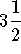,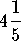, and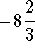are examples of compound fractions.• To manipulate compound fractions, just convert them to simple fractions and follow rules 1 through 23 for simple fractions.

Example 1: Convertto a simple fraction.
Answer.can be written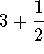. Write 3 as the fraction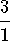. Nowcan be written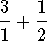and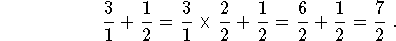• To convert a simple fraction to a compound fraction, the numerator must be larger than the denominator. Separate the whole number first.

Example 2: Convert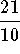to a compound fraction.

Answer. Rewriteas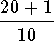. We have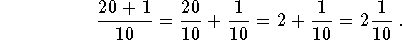• To add or subtract two compound fractions, convert the fractions to simple fractions and follow the steps you use to add or subtract two simple fractions.

Example 3: Calculate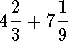.

Solution We have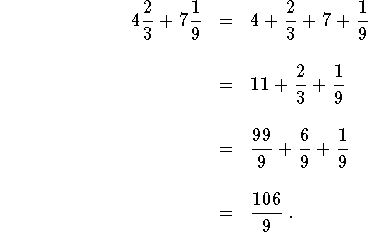• To multiply or divide two compound fractions, convert the fractions to simple fractions and follow the steps you use to multiply or divide two simple fractions.

Example 4: Calculate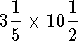.

Solution. We have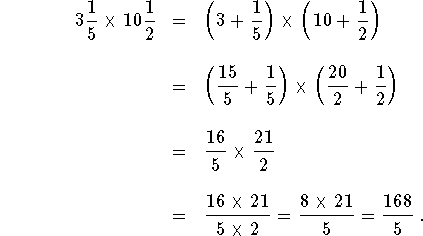### Work the following problems and click on Answer if you would like to check your answer and/or review the solution.

Problem 1:
Convert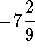to a simple fraction.

Problem 2:
Rewrite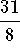as a compound number.

Problem 3:
Add the following numbers and convert the answer to a simple fraction: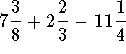.

Problem 4:
Multiply the following numbers and convert the answer to a simple fraction: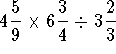.[Simple Fractions] [Complex Fractions] [Compound Fractions]
[Decimals] [Percentage] [Rules]

[Algebra] [Trigonometry] [Complex Variables]S.O.S MATHematics home page

Do you need more help? Please post your question on our S.O.S. Mathematics CyberBoard.Author: Nancy Marcus